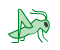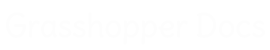﻿ Tween Through Surfaces - Pufferfish - Component for Grasshopper | Grasshopper DocsCommunity documentation for Grasshopper add-ons & pluginsColor
 Curve
 Domain
 List
 Mesh
 Number
 Plane
 Point
 SubD
 Surface
 Transform
 Twisted Box
 Vector

# Tween Through Surfaces

## Inputs

NameIDDescriptionType
SurfacesSSet of surfaces to tween throughSurface
FactorFTween factor (0.0 = Surface at S start, 1.0 = Surface at S end), unless Normalized is FalseNumber
NormalizedNIf False, the rules for factor are (0.0 = Surface at index 0, Index number of last surface = Surface at last index) The tween factor range from one surface to the next surface in the list is: Index number of a surface = the surface at that index, Index number of the next surface = the next surface in the list, factors between the surface index numbers will be the corresponding tweens. For instance: A tween factor of 2.5 will result in a tween surface halfway between the surface at index 2 and the surface at index 3.Boolean
EqualizedEIf True, tween distribution is evaluated along the tween path without considering the locations of the input surface setBoolean
WeightedWIf True, tween distribution is weighted by the input surface set and the tween path curvatureBoolean
LoopbackLIf True, allow the tween path to continue from the end back to the start as a closed loop (if Normalized is False, increase the tween factor range maximum by 1)Boolean
DegreeDCurvature degree of the tween path, degree should be a positive odd numberInteger
Interpolation TypeIDetermines how the tween path is interpolated 0 = Linear 1 = Chord 2 = Square Root 3 = UniformInteger
RefitROptional Refit match method. (No Integer or 0 = Off, Integer greater than 0 = On and surface degree of refit) If an integer greater than zero, Refit match method is used if possible. When input surfaces are refit their control points are redistributed, added to, and removed from based on the surfaces curvature and the input integer degree, while trying to maintain their shapes. Refit results in tighter shaped tweens, with curvature based control point distribution.Integer
Point SamplePOptional Point Sample match method. (No Integer or 0 = Off, Integer greater than 0 = On and amount of sample points) If an integer greater than zero, Point Sample match method is used. When input surfaces are sampled their control points are recreated by equally dividing the surface by the input integer point count. Point Sample results in looser shaped tweens, with uniform control point distribution.Integer

## Outputs

NameIDDescriptionType
TweenTResulting tween surfaceSurface

## Video Tutorials

Site design © Robin Rodricks.   Pufferfish and associated data © 2023 Michael Pryor.
Rhinoceros and Grasshopper are registered trademarks of Robert McNeel & Associates.  Hosted by GitHub